## RD Sharma Class 10 Solutions Chapter 11 Trigonometric Identities Ex 11.2

These Solutions are part of RD Sharma Class 10 Solutions. Here we have given RD Sharma Class 10 Solutions Chapter 11 Trigonometric Identities Ex 11.2

Other Exercises

Question 1.
If cos θ = $$\frac { 4 }{ 5 }$$ , find all other trigonometric ratios of angle θ
Solution: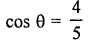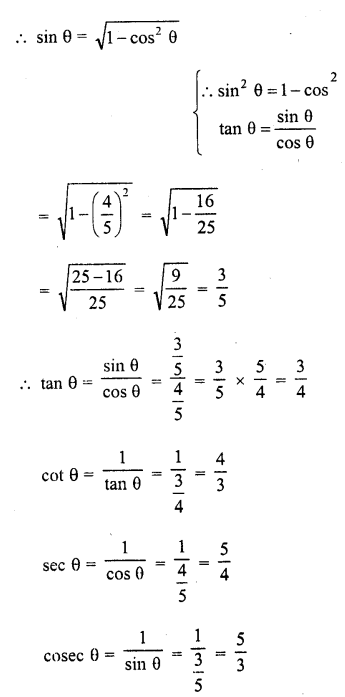Question 2.
If sin θ = $$\frac { 1 }{ \sqrt { 2 } }$$ , find all other trigono­metric ratios of angle θ
Solution: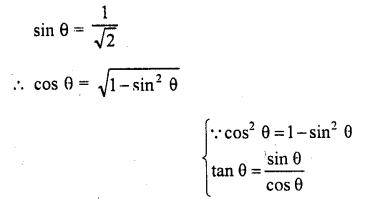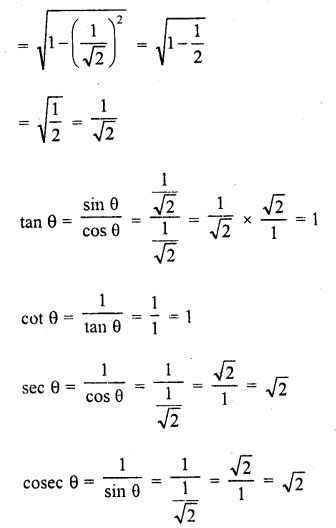Question 3.
If tan θ = $$\frac { 1 }{ \sqrt { 2 } }$$, find the value of $$\frac { { cosec }^{ 2 }-{ sec }^{ 2 } }{ { cosec }^{ 2 }+cot^{ 2 } }$$
Solution:
<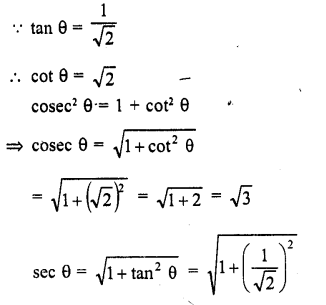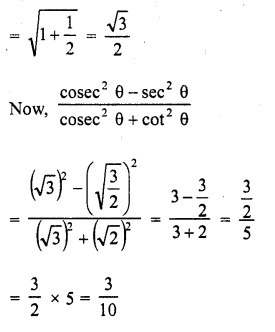Question 4.
If tan θ = $$\frac { 3 }{ 4 }$$, find the value of $$\frac { 1-cosheta }{ 1+cosheta }$$
(C.B.S.E. 1995)
Solution: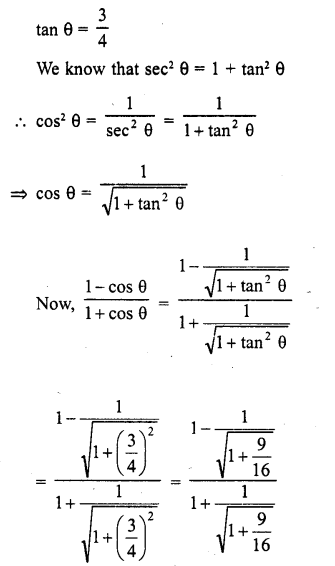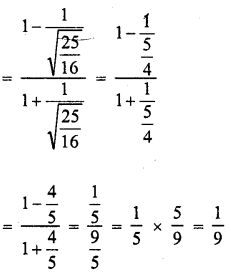Question 5.Solution: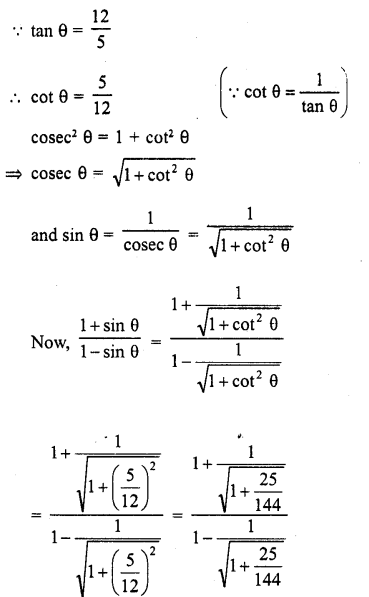Question 6.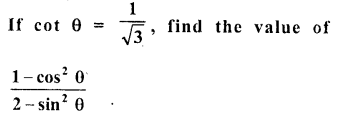Solution: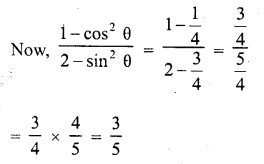Question 7.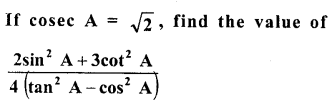Solution: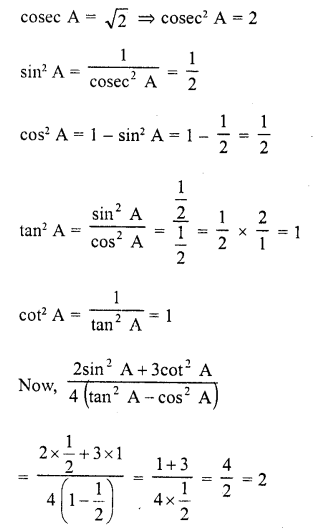Question 8.Solution: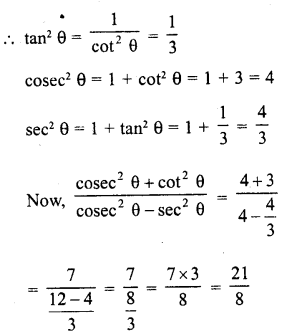Question 9.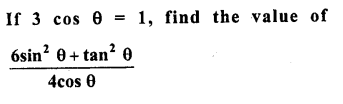Solution: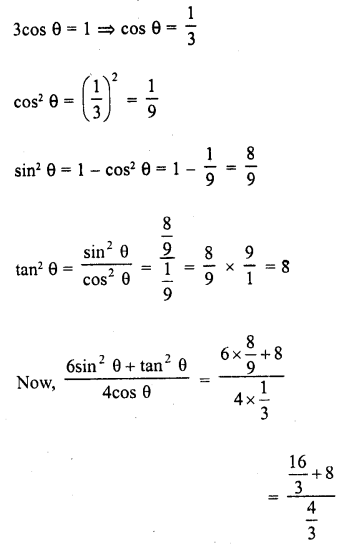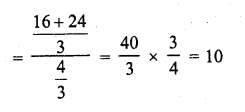Question 10.
If $$\sqrt { 3 }$$ tan θ = 3sin θ , find the value of sin2 θ  – cos2 θ .          (C.B.S.E. 2001)
Solution:Question 11.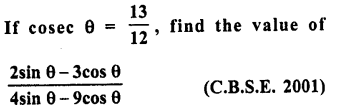Solution: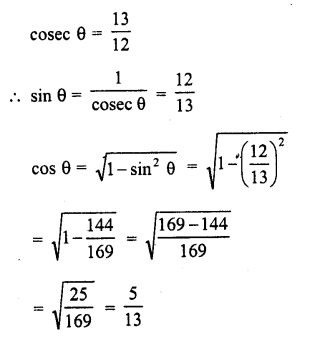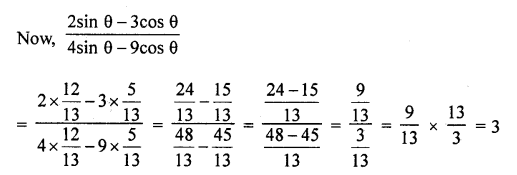Question 12.
If sin θ + cos θ = $$\sqrt { 2 }$$cos (90° – θ), find cot θ
Solution: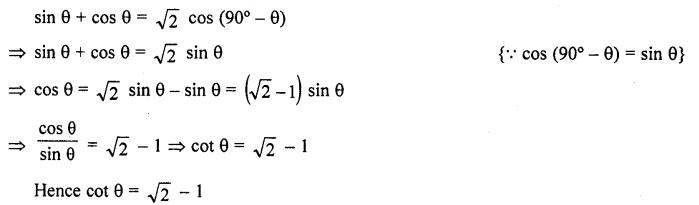Question 13.
If 2sin2 θ – cos2 θ = 2, then find the value of θ. [NCERT Exemplar]
Solution:Question 14.
If $$\sqrt { 3 }$$ tan θ-1=0, find the value of sin2 – cos2 θ. [NCERT Exemplar]
Solution: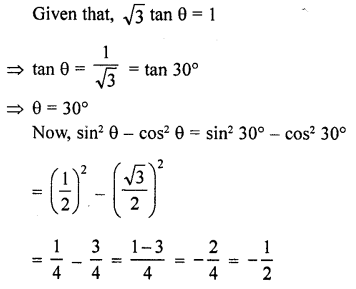Hope given RD Sharma Class 10 Solutions Chapter 11 Trigonometric Identities Ex 11.2 are helpful to complete your math homework.

If you have any doubts, please comment below. Learn Insta try to provide online math tutoring for you.